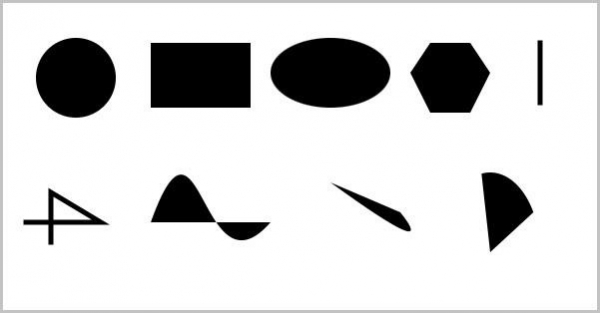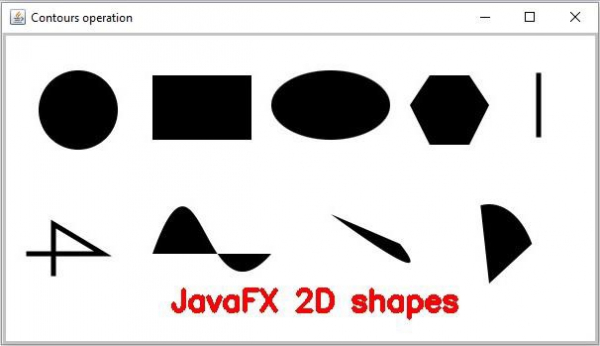# How to add text to an image using Java OpenCV library?

You can add text to an image using the putText() method of the org.opencv.imgproc.Imgproc class. This method renders the specified text in the given image. It accepts −

• An empty mat object to store the source image.

• A string object to specify the desired text.

• A Point object specifying the position of the text.

• Integer constant specifying the font of the text.

• scale factor that is multiplied by the font-specific base size.

• A Scalar object specifying the color of the text.

• An integer value specifying the color of the text

## Example

import org.opencv.core.Core;
import org.opencv.core.Mat;
import org.opencv.core.Point;
import org.opencv.core.Scalar;
import org.opencv.highgui.HighGui;
import org.opencv.imgcodecs.Imgcodecs;
import org.opencv.imgproc.Imgproc;
public static void main(String args[]) throws Exception {
//Reading the contents of the image
String file ="D:\Images\shapes.jpg";
//Preparing the arguments
String text = "JavaFX 2D shapes";
Point position = new Point(170, 280);
Scalar color = new Scalar(0, 0, 255);
int font = Imgproc.FONT_HERSHEY_SIMPLEX;
int scale = 1;
int thickness = 3;
Imgproc.putText(src, text, position, font, scale, color, thickness);
//Displaying the resultant Image
HighGui.imshow("Contours operation", src);
HighGui.waitKey();
}
}

## Input Image## Output